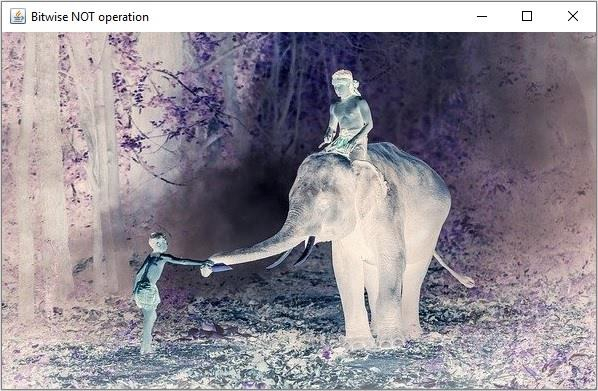# How to perform Bitwise Not operation on images using Java OpenCV?

You can compute the bitwise conjunction between two images using the bitwise_not() method of the org.opencv.core.Core class.

This method accepts two Mat objects representing the source and destination matrices, calculates the inverse of each element in the source matrix and stores the result in the destination matrix.

## Example

import org.opencv.core.Core;
import org.opencv.core.Mat;
import org.opencv.highgui.HighGui;
import org.opencv.imgcodecs.Imgcodecs;
public class BitwiseNOTExample {
public static void main(String args[]) throws Exception {
String file ="D://images//elephant.jpg";
HighGui.imshow("Grayscale Image", src);
//Creating an empty matrix to store the result
Mat dst = new Mat(src.rows(), src.cols(), src.type());
//Applying bitwise not operation
Core.bitwise_not(src, dst);
HighGui.imshow("Bitwise NOT operation", dst);
HighGui.waitKey();
}
}

## Input Image## Output

On executing, the above program generates the following windows −